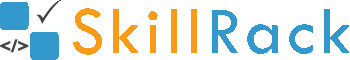Find the square root of an integer without using any in-built function4 months ago

How to find the square root of an integer without using any in-built function?

Using Newton Raphson method we can find the square root of an integer without using any in-built function.

The C solution is given below.

#include<stdio.h>
//Newton Raphson method to find square root without using in built function

double absolute(double val){
return val < 0 ? -val:val;
}

double findSquareRoot(int N){
const double diff = 0.00001;
double sqRoot = 1.0;
while( absolute(N - (sqRoot*sqRoot)) >= diff){
sqRoot = (N/sqRoot + sqRoot)/2.0;
}
return sqRoot;
}

int main()
{
int N;
scanf("%d",&N);
printf("\n%.5lf",findSquareRoot(N));
return 0;
}